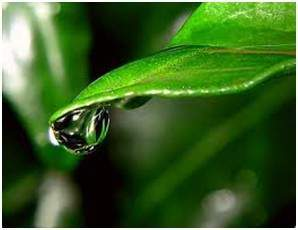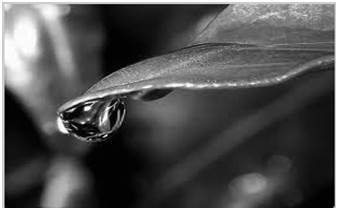## Wednesday, January 29, 2020

### Grayscale to RGB conversion

we will convert an color image into a grayscale image. There are two methods to convert it. Both has their own merits and demerits. The methods are:
• Average method
• Weighted method or luminosity method
• using skimage

## Average method

Average method is the most simple one. You just have to take the average of three colors. Since its an RGB image, so it means that you have add r with g with b and then divide it by 3 to get your desired grayscale image.
Its done in this way.
Grayscale = (R + G + B / 3)

For example:If you have an color image like the image shown above and you want to convert it into grayscale using average method. The following result would appear.### Explanation

There is one thing to be sure, that something happens to the original works. It means that our average method works. But the results were not as expected. We wanted to convert the image into a grayscale, but this turned out to be a rather black image.

### Problem

This problem arise due to the fact, that we take average of the three colors. Since the three different colors have three different wavelength and have their own contribution in the formation of image, so we have to take average according to their contribution, not done it averagely using average method. Right now what we are doing is this,
33% of Red, 33% of Green, 33% of Blue
We are taking 33% of each, that means, each of the portion has same contribution in the image. But in reality thats not the case. The solution to this has been given by luminosity method.

## Weighted method or luminosity method

You have seen the problem that occur in the average method. Weighted method has a solution to that problem. Since red color has more wavelength of all the three colors, and green is the color that has not only less wavelength then red color but also green is the color that gives more soothing effect to the eyes.
It means that we have to decrease the contribution of red color, and increase the contribution of the green color, and put blue color contribution in between these two.
So the new equation that form is:
New grayscale image = ( (0.3 * R) + (0.59 * G) + (0.11 * B) ).
According to this equation, Red has contribute 30%, Green has contributed 59% which is greater in all three colors and Blue has contributed 11%.
Applying this equation to the image, we get this
Original Image:Grayscale Image:### Explanation

As you can see here, that the image has now been properly converted to grayscale using weighted method. As compare to the result of average method, this image is more brighter.

### using skimage

```import numpy as np
import matplotlib.pyplot as plt

from skimage import data
from skimage.color import rgb2gray

original = data.astronaut()
grayscale = rgb2gray(original)

fig, axes = plt.subplots(1, 2, figsize=(8, 4))
ax = axes.ravel()

ax.imshow(original)
ax.set_title("Original")
ax.imshow(grayscale, cmap=plt.cm.gray)
ax.set_title("Grayscale")

fig.tight_layout()
plt.show()```